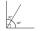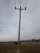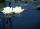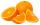Equation - problems - page 51

1. A residentialA residential colony has a population of 5400 and 60 litres of water is required per person per day. For the effective utilization of rain water, they constructed a water reservoir measuring 48m × 27m × 25m to collect the rain water. For how many days, the
2. Seven timesWhich number seven times is just as higher as 27, how much is smaller than 29?
3. Father and sonWhen I was 11, my father was 35 years old. Today, my father has three times more years than me. How old is she?
4. 2 pipes2 pipes can fill a tank in 35 minutes. The larger pipe alone can fill the tank in 24 minutes less time than the smaller pipe. How long does each pipie take to fill the tank alone?
5. Number with onesThe first digit of the number is 1, if we move this digit to the end we get a 3 times higher number, which is the number?
6. Average heightThe average height of all pupils is 162 cm. The class teacher's height is 178 cm. The average height of all (teacher and all pupils) is 163 cm. Calculate the number of pupils in the class.
7. Three peopleThree people start doing a work at a same time. The first worked only 2 hours. The second ended 3 hours before the end. On an individual basis, it would take the first time to do the work 10 hours, second 12 hours and 15 hours third. How many hours did it.
8. Dropped sheetsThree consecutive sheets dropped from the book. The sum of the numbers on the pages of the dropped sheets is 273. What number has the last page of the dropped sheets?
9. 13 ticketsA short and long sightseeing tour is possible at the castle. Ticket for a short sightseeing circuit costs CZK 60, for a long touring circuit costs CZK 100. So far, 13 tickets have been sold for 1140 CZK. How much did you pay for tickets for a short tour?
10. The touristThe tourist traveled 190km in 5 hours. Part of the journey passed at 5 km/h. The rest he went by bus at a speed of 60 km/h. How long has a bus gone?
11. Two trainsThere were 159 freight wagons on the railway station creating 2 trains. One had 15 more wagons than the other. How many wagons did each train have?
12. Complementary angles 2Two complementary angles are (x+4) and (2x - 7) find the value of x
13. Substitutionsolve equations by substitution: x+y= 11 y=5x-25
14. Iron poleThe iron pole is in the ground 2/5 of its length, partly above the ground 1/3 is yellow, and the unpainted section is 6 m long. How long is the entire column?
15. PlayingHow long have we trained on the pitch when we know that the warm-up took 10 minutes, we trained passes for one-third of the time and we played football half the time?
16. Equation 29Solve next equation: 2 ( 2x + 3 ) = 8 ( 1 - x) -5 ( x -2 )
17. Water liliesWater lilies are growing on the pond and their number is doubled every day. The whole layer is covered in 12 days. How many days will it cover 8 layers?
18. The largerThe larger of two numbers is nine more than four times the smaller number. The sum of the two numbers is fifty-nine. Find the two numbers.
19. Two shipsThe distance from A to B is 300km. At 7 am started from A to B a ferry with speed higher by 20 km/h than a ship that leaves at 8 o'clock from B to A. Both met at 10h 24min. Determine how far they will meet from A and when they reach the destination.
20. OrangesMother divided her three children's oranges in a ratio of 6:5:4. Two children gave 45 oranges. How many oranges were there?

Do you have an interesting mathematical problem that you can't solve it? Enter it, and we can try to solve it.

To this e-mail address, we will reply solution; solved examples are also published here. Please enter e-mail correctly and check whether you don't have a full mailbox.

Do you have a linear equation or system of equations and looking for its solution? Or do you have quadratic equation?